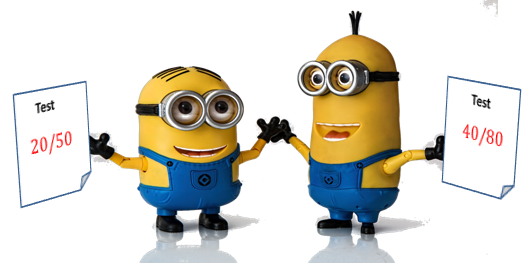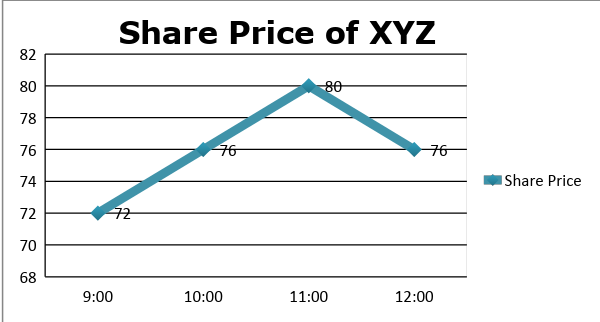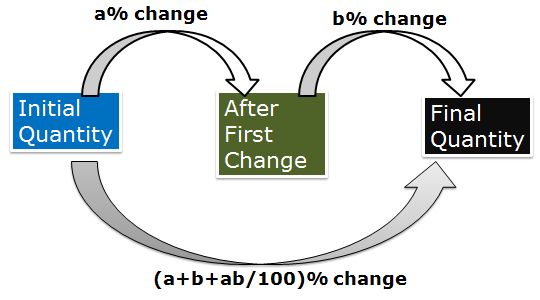# Arithmetic

#### Simple Interest & Compound Interest - 1

Percentages: 1

Percentages:

Percentage is a mathematical tool that is used to compare values and to quantify the changes (whether increase or decrease) taking place in values of quantities. Even though it is a mathematical operator, it finds its application in almost every field of study. This chapter holds the key to the Arithmetic section and problems on Data Interpretation. Thus, understanding and having a good command over this chapter is a prerequisite if one aspires to score good marks in any aptitude test.

What do we mean by percentage?

Percent means per hundred, i.e. it is a fraction whose denominator or whose base is 100. It can also be viewed as a ratio expressed as a fraction of 100. To understand more clearly, let’s take an example.Two students A and B appeared for two different test – T1 and T2. A scored 20 marks out of a total of 50 marks in T1 and B scored 40 out of a total of 80 in T2. Which student has fared better? This is difficult to assess as both these tests have a different total number of marks. Now to make things simpler we bring both these tests to a same base or in other words we extrapolate the marks of students if these tests had a total of 100 marks. So if A scored 20 out of 50, then he would have scored 40 marks out of 100. Similarly B would have scored 50 marks if the total marks were 100. Now it is easy to make a comparison between the students. Definitely B  has fared better.

This is precisely the procedure/process applied in calculating percentages. So percentage simply means the value of a quantity when it is compared to a base value which is 100. Percent is a dimensionless quantity and is usually denoted by ‘%’ symbol.

If Tom had Rs 90 before going to the market and he spent Rs 30 on buying groceries, then we can say that he spent 30/90 i.e 1/3rd of his money. In terms of percentage we can say that out of Rs 90, he spent RS 30, so if he had Rs 100 then he would have spent (30/90)*100 = 33.33% of his money.

If it were given that Tom spent 20% of his money on groceries and he took Rs 80 along with him, then how much would he have spent on buying groceries?

This can again be understood as- If Tom had Rs 100, he would have spent Rs 20 on groceries, i.e. 1/5th . So if he had Rs 80, then he would have spent 1/5th of that i.e. Rs 16 on groceries.

Hope the idea is clear now. There are basically two ways of asking a questionFractions Percentage Equivalent Fractions Percentage Equivalent Fractions Percentage Equivalent 1/1 100 % 1/11 9111% or 9.09% 1/32 318% or 3.125% 1/2 50% 1/12 813% or 8.33% 3/25 12% 1/3 3313% or 33.33% 1/13 7913% or 7.69% 3/20 15% 1/4 25% 1/14 717% or 7.14 % 2/15 1313% or 13.33% 1/5 20% 1/15 623% or 6.66% 3/8 3712% or 37.5 % 1/6 1623% or 16.66% 1/16 614% or 6.25% 2/3 6623% or 66.66% 1/7 1427% or 14.28% 1/17 51517% or 5.88% 5/8 6212% or 62.5% 1/8 1212%  or 12.5% 1/18 559% or 5.55% 7/8 8712% or 87.5% 1/9 1119% or 11.11% 1/19 5519% or 5.26% 2/5 40% 1/10 10% 1/20 5% 1/30 313% or 3.33%

Percentage Increase/Decrease:

Percentage is also used to quantify changes in value. Very often we express the change in value of a quantity with respect to the initial value in terms of percentage. Let’s take an example. The share price of a single share of company XYZ at different times in a particular day is as shown in the table:

 Share Price (in Rs) 72 76 80 76 Time 09:00 10:00 11:00 12:00So, from 9:00-10:00 the value of share increased from 72 to 76. i.e. an increase of 4 Rs in the price. But if we want to know the percentage change in the price of share from 9:00-10:00, then we also have to consider the price (base) at which the change occurred. i.e. the change of 4 Rs occurred at the price (72) which was at 9:00.Multiplying Factor:

Let us say Raghav had 120 marbles last month and this month they increased by 20%. So, how many marbles does he have now?

This increase of 20% is on 120 marbles, so increase = 20% of 120 = 24

Final number of marbles = 120 + 24 = 144

This entire procedure can be done in one step with the help of multiplying factor.

Final quantity = Initial + Increase

Final = 120 + 20% of 120 = 120 + 0.2*120 = 120(1+0.2) = 1.2*120

This 1.2 is called the multiplying factor

Similarly if the marbles would have decreased by 10%, then

Final = Initial – Decrease = 120 -10% of 120 = 120 (1-0.1) = (0.9)*120

So here the multiplying factor is 0.9. We simply need to multiply the initial value with the multiplying factor to obtain the final or new value.Initial Value Percentage Change Multiplying Factor Final value 80 30%  Increase 1.3 80*1.3 = 104 80 15%  Decrease 0.85 80*0.85 = 68 80 37.5%  Increase 1.375 80 * 1.375 = 110 80 12%  Decrease 0.88 80 * 0.88 = 70.4 80 6.25% Decrease 0.9375 80* 0.9375 = 75 80 17.5% Increase 1.175 80* 1.175 = 94Example 4: From my salary, 20% was deducted as house rent. 15% of the remaining is spent on food, 10% of the balance on clothing, now I am left with 3000. Find my salary.

A. 4000                     B. 4800           C.4902                   D.5117

Solution:  Let the salary be x Rs

After paying the house rent, salary left = 80% of x  or 0.8x

Now further 15% of the remaining is spent on food, so amount left = 0.85*0.8x

Further 10% of the remaining is spent on clothing, so amount left = 0.9*0.85*0.8x

which is equal to 3000,

So 0.9*0.85*0.8x = 3000

Or x = 4902 (approximately)

Example 5: Two students appeared in an examination. One scored 9 marks more than the other and his marks were 60% of the total of their marks. Find out the individual marks

A. 36,45         B. 9,18     C.18,9      D. 27,18

Solution:  Let the marks scored by the first student be x and so the marks scored by the second student becomes x+9

Given that (x+9) = 60% of (x+x+9)

Or x + 9 = 3/5(2x+9)

5x + 45 = 6x + 27

Or  x = 18

So, the marks scored by the two students are 18 and 27.

Example 6: Tom scored 50% more marks than Harry and Dick scored 20 % more marks than Harry, then how much percent more marks did Tom score than Dick?

Solution: If Harry scored 100 marks, then marks scored by Tom = 1.5*100 = 150

Similarly marks scored by Dick = 1.2*100 = 120

So, Tom’s score is more than Dick’s by 150-120/120*100=25%

In the same question if it were asked by how much percentage is Dick’s marks less than Tom’s marks, then the answer would not have been 25% because here the base would be Tom’s marks as the comparison is made with his marks

So, answer in this case would have been 150-120/150*100=20%

Example 7: A’s share in the profit is 25% more than that of B, whose share is 20% more than that of C. By how much percent is A’s share greater than C’s?

A. 20.35           B. 33.33 C. 41.60            D. None of theseQuestions on Percentage Change in Price, Expenditure and Consumption:

These type of problems are very frequently witnessed by students in all sort of exams related to aptitude. These problems involve the concept of percentage change which can be very easily applied if we have a clear understanding of the concepts.

There is a basic equation that we need to learn, i.e.

Price * Consumption = ExpenditureIt means if the price of a commodity is increased, then to keep the same expenditure, we need to reduce the consumption.

Similarly if the price goes down then we have to increase the consumption so as to keep the expenditure same.

In case the price goes up and the consumption remains the same, then expenditure will surely increase.

Or if the price goes down and the consumption remains the same, then expenditure will go down.

In short we can say that price is directly proportional to expenditure, consumption is directly  proportional to expenditure and the price and consumption are inversely proportional; to each other.

Let’s solve a few problems on the concept above:

Example 8: If the price of rice increase by 20%, then by how much percentage , the consumption of rice must be reduced by a family so as to keep the same expenditure?Example 9: The price of kerosene decreased by 20% while the consumption of it increased by 10% in a family. What is the percentage change in the expenditure on kerosene by the family?The same concept can be applied in all the cases where product of two parameters is equal to the third. For example

Length * Breadth = Area of the rectangle

Speed * Time = Distance

Number of men * Time = Work done

And so on

Example 10: Hanu goes to karate class from his home every day and leaves his home at 5 P.M. reaching the class by 5:30. One day he increased his speed by 20%. At what time will he reach his class that day?

Solution: Here the distance between the karate class and home remains the same. So speed and time are inversely proportional to each other.

As the speed increases by 20%, so Sf/Si = 1.2/1 or 6/5

Speed * Time = Distance (constant in this case)

We can say that Tf/Ti = 5/6 ( Speed and Time are inversely proportional)

So the final time taken would be 5/6 times the in initial time taken

Initial time taken by hanu = 30 minutes

Now, the time taken would be (5/6)* 30 = 25 minutes.

So, Hanu would reach his karate class at 5:25 P.M.

Example 11: If the price of an entity is increased by 25%, but the budget increases by only 8%, by how much percent should the consumption be cut down?

A. 12%              B. 13%        C. 13.6%            D. 9.09%Example 12: Ankit goes to a shop with an amount of Rs 300 to buy apples with the entire amount for his mother. But the shopkeeper told him that the prices of apples have been reduced by 40%. Now with the reduced prices, he was able to buy 10 more apples with the same amount. What is the new price per apple?

Solution:  Here the total amount remains same, i.e Rs 300. Now the prices reduced by 40%, so Pf/Pi = 6/10 = 3/5 . So the final quantity of apples bought would become 5/3 times of the original.

If the original quantity of apples bought were 3x, then final quantity would be 5x.

So, extra apples = 2x = 10. So, x = 5

Thus, final number of apples bought = 5*5 = 25

Total amount = Rs 300

So, new price per apple = 300/12 = Rs 25

Successive Change

Let us say we have a pen whose price is Rs 100. If the price is increased by 10%, the new price becomes 1.1*100 = 110. Now again if the price is increased by 20%, then the new price would be 1.2*110 =  Rs 132.

We can say that 100 was first increased by 10% and then by 20% i.e. the original price was successively increased by 10% and 20%. If we compare the final price with the original price, then

Percentage Change= 132-100/100*100=32%

So, we can say that  a successive increase of 10% and then 20% is equivalent to a direct change of 32%. There is a formula to directly find a single equivalent percentage change

If a quantity is first increased by a% and then by b%, then it is equivalent to a single change of (a + b + ab/100) %.Now if a quantity is first increased by a% and then decreased by b%, then put –b instead of b to get the required percentage change. So, formula in this case would become

Equivalent change = (a-b-ab/100)

Similarly if it is decreased by a% and then increased by b%, then use –a instead of a to get the required result.

If both the times the quantity is decreased, then use –a and –b instead of a and b to get the result. Of course if the final result is negative the n it would mean a decrease.

For example if a quantity is first decreased by 10% and then by 20%, then

Equivalent change = -10  -20 + (10*20)/100 = -28%

So, the quantity has decreased by 28%.

Example 13: The length of a rectangle is increased by 12.5% while its breadth is decreased by 20%. How much is the percentage change in area of the rectangle?

Solution: Here we can use the concept of successive change to find out the equivalent change in area of the rectangle.

Length is increased by 12.5%, so a = 12.5

Breadth is decreased by 20%, so b = -20

Equivalent change = 12.5 -20-  -12.5*20/100 = -10%

So the area of the rectangle reduces by 10%.Let us solve example 11 using successive change formula:

Here a = 25% , equivalent change= c% = 8% , consumption change = b = ?

c = a+ b + ab /100

8 = 25 + b + 25b/100

Or 5b/4 = -17     or b = -13.6%

Thus, consumption must be reduced by 13.6%.

Example 14: A shopkeeper offers three successive discounts of 30%, 20% and 10% on the marked price of a watch. What single discount in percentage is equivalent to these three successive discounts?

Solution: Till now we have seen the formula for only two successive percentage changes. For three or more the method is to combine the first and the second and calculate their equivalent change. Now combine this with the third percentage using the formula to calculate the overall percentage change.

Here a = -30% , b =- 20% and c = -10%  (As all are discounts)

Let d = -30 -20 + (30*20)/100  = -44%

Now we will solve with d and c

Final Equivalent Discount = -44 -10 + (44*10)/100 = -49.6%

Thus, a successive discount of 30%,20% and 10% is equivalent to a single discount of 49.6%.

Another way of solving this problem is – Let the marked price of watch be Rs 100

So after first discount of 30%, the price become 0.7*100 = 70

After second discount of 20%, the price becomes 0.8*70 = 56

After third discount of 10%, the price becomes – 0.9*56 = 50.4

So overall discount offered = 100-50.4 = 49.6

% Discount = 49.6100*100=49.6%

Example 15: If the radius of a sphere is increased by 20%, by how much does the volume of its hemisphere increase?